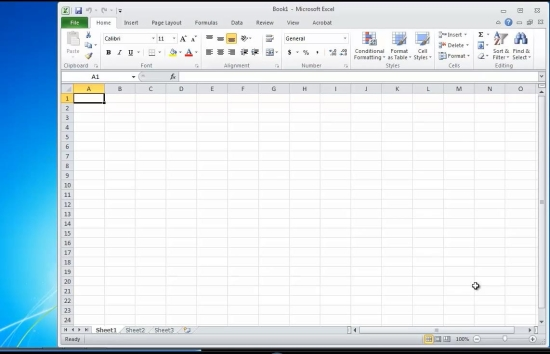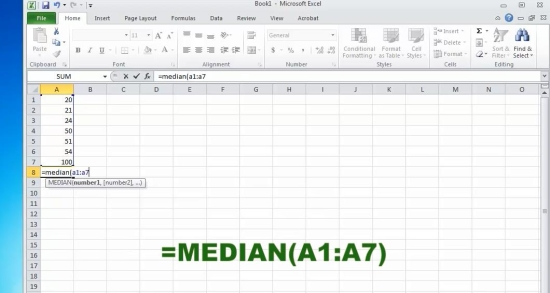## How to Calculate the Median in Excel

In this tutorial you will learn how to calculate the median in Excel

Calculating the mean from a set of numbers is done by the formula: Median (number set).

This means you need a set of numbers to calculate the median for.

Let’s create it:

Prerequisites: Microsoft Excel

Step 1. Launch Excel and start a new file from File- New.Step 2. Fill several rows with the various values you want to calculate the median for.

Step 3. Select the cell where you want to add the calculation.

Type: = median(AAxx: AAyy), where

AA is the column that has your numbers aligned, and xx and yy are the beginning and the end of your series of numbers. Hit Enter.Result: Your median value is displayed.

Congratulations, you have learnt how to calculate the median in Excel.